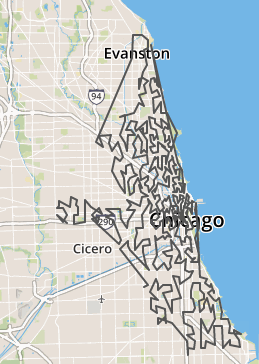# A Divvy tour using a Space Filling Curve

In a couple of previous posts, I reviewed the concept of using space filling curves as a heuristic for producing decent solutions for the traveling salesman problem (TSP). The attraction of this approach to me was the simplicity of implementation. Specifically, the ability to avoid the calculation of the distance matrix is particularly appealing.

I thought I’d show an implementation here of a toy problem I have addressed elsewhere on this site. In 2015, I did a fun little project of applying the TSP to visiting every station in Chicago’s Divvy bike sharing program.

I’m a small consulting entity, so I don’t have commercial access to the Google Maps API and thus am capped to 2500 calls a day. At the time I did the 2015 Divvy project, there were 300 stations in the system , which required the calculation of 300×300 = 90,000 distance matrix entries. (OK, full disclosure: I assumed symmetry travelling between any two stations, so I really only calculated 45,000 distances using the API). It’s not a lot of actual work once it is automated, but that still required 18 days of calendar time to collect those numbers.
Fast forward to 2017 and there are now nearly 600 stations, so I’d be looking at 600×600 = 360,000 or about 144 calendar days;
I’ve got better ways to spend the next 5 months. So do you.

Another concern as the number of “stations” in the problem rises is the computing power necessary to come up with a decent solution. In the 2015 project, I used a nearest neighbor approach, which was feasible for a toy probelm with 300 stations, but would get more computationally intensive as the number of stations visited rises or the algorithm used becomes more sophisticated.

So enough of my kvetching and onto the problem at hand. I wanted to use a space filling curve to try and calculate a fairly efficient route to visit all the approximately 600 Divvy stations. The steps were still similar to the 2015 project , if slightly scaled up due to the increased number of stations. I downloaded the stations and their associated latitudes and longitudes and loaded them into a MySQL database. The code to produce the tour follows at the end of the post. For here, I’ve just show the GEOJSON representation, represented on the great site geojson.io .A couple of thoughts regarding this:

1. It would be interesting to compare the distance of this versus a more standard appoach to calculating a tour. But that would require me to calculate that distance matrix, which I’m trying to avoid ! I may try this with the 2015 stations, where I have everything in hand to do the comparison
2. The representation shown is different from my 2015 project, where I showed a turn by turn representation rather than straight line
3. I would guess those long legs in the NW part of the tour are unavoidable. As the Divvy program expanded , the stations have gone farther from the city center , but are also more sparse outside the city center. It’s sort of like building a baseball roadtrip. No matter how you build it, there will always be some long leg drive to get to Seattle !

Here is the Python code to create the GEOJSON representation from the database containg the stations and the lats and longs.

```# Create the GEOJSON file

import json
from shapely.geometry  import  Point,mapping
import MySQLdb
from math import floor,ceil

db = MySQLdb.connect("127.0.0.1","root","xxxxxxx","divvy2017")
#db.autocommit(True)
div_cursor = db.cursor()

div_sql = """select id,divvy_id,name,latitude, longitude from stations
;"""

gjson_dict={}
gjson_dict["type"]= "FeatureCollection"
feat_list = []
type_dict = {}
ls_dict = {}
prop_dict = {}
coord_list = []
type_dict["type"]= "Feature"

ls_dict["type"]="LineString"

#Maxint is the granularity of the grid ....
maxint = 200

#Define a bounding box; these are just the extemes of lat and long for all stations
lat_min = 41.736646
lat_max = 42.063999
lng_min = -87.80287
lng_max = -87.54938625

lat_len = lat_max - lat_min
lng_len = lng_max - lng_min

#Initialize
ind_dict = {}
ll_dict = {}

div_cursor.execute(div_sql)
div_list= div_cursor.fetchall()

for div in div_list:

dname = div
dlat = float(div)
dlng = float(div)

# Find the closest spot on this grid
dlat_ind = round(maxint*(dlat - lat_min)/lat_len,0)
dlng_ind = round(maxint*(dlng - lng_min)/lng_len,0)

# Calculate sierp_ind
s_ind = sierp_index(int(dlat_ind),int(dlng_ind),maxint)
ll_dict[dname] = [dlat,dlng]
ind_dict[dname] = s_ind

#Building the GEOJSON coordinates now that we have have the stations in trip order
for key, value in sorted(ind_dict.iteritems(), key=lambda (k,v): (v,k)):
coord_list.append([ll_dict[key],ll_dict[key]])

ls_dict["coordinates"]=coord_list
type_dict["geometry"]=ls_dict
# This is sort of a dummy.....we don;t really have any proprties or the entire curve
prop_dict["dist_miles"]= 1
type_dict["properties"]=prop_dict

feat_list.append(type_dict)
gjson_dict["features"] = feat_list

with open('c:\\DivvySFC2017\\test_geojson.json', 'w') as outfile:
json.dump(gjson_dict, outfile, sort_keys = True, indent = 4,
ensure_ascii=False)

```